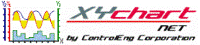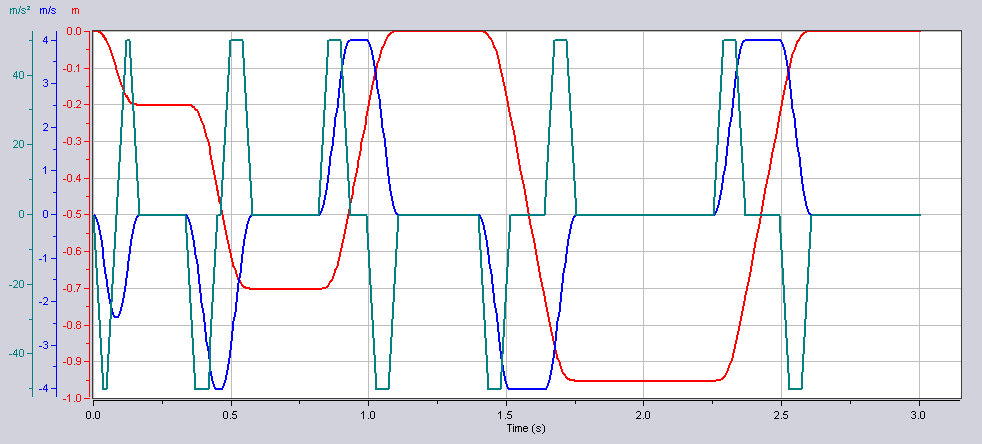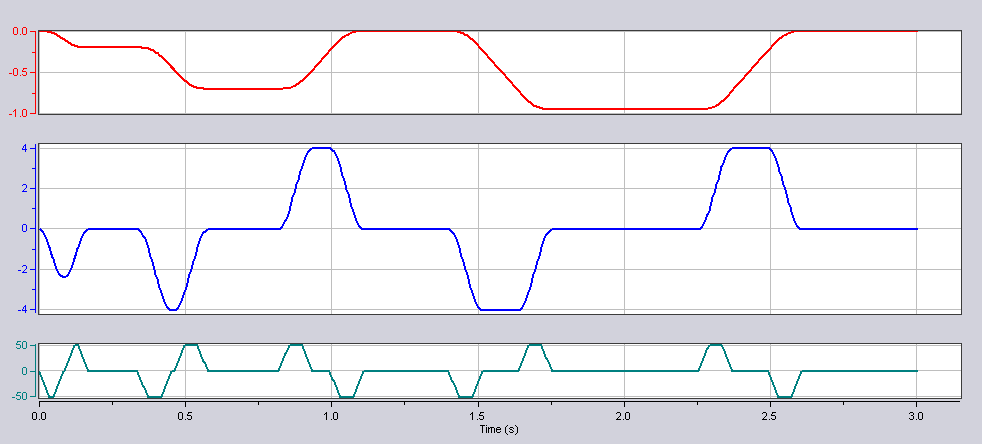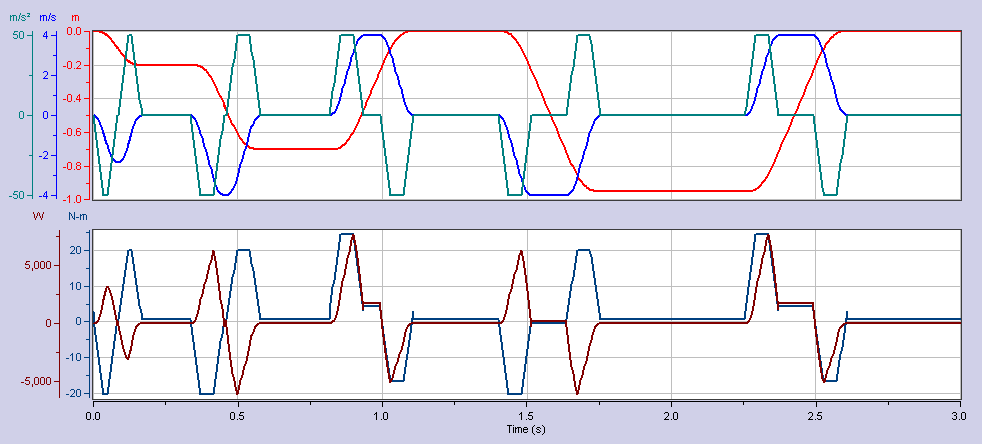XY Chart NET 3 Control Reference
Y-Scale Stacking

Y-Scale Stacking feature allows you to display one or more y-scales per plot (aka YStack).  The plots are stacked vertically with the first y-stack on top, then the second, and so on.  This feature is only available if the number of YScales (NumYScales) is greater than 1.

See examples 1, 2 and 3 below.The graphs in examples 1 & 2 display the same data.

Example 1 - Y Stacking Not Used```' XYChartNETCtl1 is the name of the XY Chart NET control instance placed on the form.

With XYChartNETCtl1
.NumYScales = 3
.NumProfiles = 3

.YStacking = False

.Profile(0).YScale = 0
.Profile(1).YScale = 1
.Profile(2).YScale = 2
End With
```
```// XYChartNETCtl1 is the name of the XY Chart NET control instance placed on the form.

XYChartNETCtl1.NumYScales = 3;
XYChartNETCtl1.NumProfiles = 3;

XYChartNETCtl1.YStacking = false;

XYChartNETCtl1.get_Profile(0).YScale = 0;
XYChartNETCtl1.get_Profile(1).YScale = 1;
XYChartNETCtl1.get_Profile(2).YScale = 2;
```
```// XYChartNETCtl1 is the name of the XY Chart NET control instance placed on the form.

XYChartNETCtl1->NumYScales = 3;
XYChartNETCtl1->NumProfiles = 3;

XYChartNETCtl1->YStacking = false;

XYChartNETCtl1->get_Profile(0)->YScale = 0;
XYChartNETCtl1->get_Profile(1)->YScale = 1;
XYChartNETCtl1->get_Profile(2)->YScale = 2;
```
Example 2 - Y-Scale Stacking Used (1x1x1)```' XYChartNETCtl1 is the name of the XY Chart NET control instance placed on the form.

With XYChartNETCtl1
.NumYScales = 3
.NumProfiles = 3

.YStacking = True
.NumYStacks = 3
.YStack(0).NumYScales = 1  'Y-Stack 0 will contain Y-Scale 0
.YStack(1).NumYScales = 1  'Y-Stack 1 will contain Y-Scale 1
.YStack(2).NumYScales = 1  'Y-Stack 2 will contain Y-Scale 2

.Profile(0).YScale = 0
.Profile(1).YScale = 1
.Profile(2).YScale = 2
End With
```
```// XYChartNETCtl1 is the name of the XY Chart NET control instance placed on the form.

XYChartNETCtl1.NumYScales = 3;
XYChartNETCtl1.NumProfiles = 3;

XYChartNETCtl1.YStacking = true;
XYChartNETCtl1.NumYStacks = 3;
XYChartNETCtl1.get_YStack(0).NumYScales = 1;  //Y-Stack 0 will contain Y-Scale 0
XYChartNETCtl1.get_YStack(1).NumYScales = 1;  //Y-Stack 1 will contain Y-Scale 1
XYChartNETCtl1.get_YStack(2).NumYScales = 1;  //Y-Stack 2 will contain Y-Scale 2

XYChartNETCtl1.get_Profile(0).YScale = 0;
XYChartNETCtl1.get_Profile(1).YScale = 1;
XYChartNETCtl1.get_Profile(2).YScale = 2;
```
```// XYChartNETCtl1 is the name of the XY Chart NET control instance placed on the form.

XYChartNETCtl1->NumYScales = 3;
XYChartNETCtl1->NumProfiles = 3;

XYChartNETCtl1->YStacking = true;

XYChartNETCtl1->NumYStacks = 3;
XYChartNETCtl1->get_YStack(0)->NumYScales = 1;  //Y-Stack 0 will contain Y-Scale 0
XYChartNETCtl1->get_YStack(1)->NumYScales = 1;  //Y-Stack 1 will contain Y-Scale 1
XYChartNETCtl1->get_YStack(2)->NumYScales = 1;  //Y-Stack 2 will contain Y-Scale 2

XYChartNETCtl1->get_Profile(0)->YScale = 0;
XYChartNETCtl1->get_Profile(1)->YScale = 1;
XYChartNETCtl1->get_Profile(2)->YScale = 2;
```
Example 3 - Y-Scale Stacking Used (3x2)```' XYChartNETCtl1 is the name of the XY Chart NET control instance placed on the form.

With XYChartNETCtl1
.NumYScales = 5
.NumProfiles = 5

.YStacking = True
.NumYStacks = 2
.YStack(0).NumYScales = 3  'Y-Stack 0 will contain Y-Scales 0, 1 and 2
.YStack(1).NumYScales = 2  'Y-Stack 1 will contain Y-Scales 3 and 4

.Profile(0).YScale = 0
.Profile(1).YScale = 1
.Profile(2).YScale = 2
.Profile(3).YScale = 3
.Profile(4).YScale = 4
End With
```
```// XYChartNETCtl1 is the name of the XY Chart NET control instance placed on the form.

XYChartNETCtl1.NumYScales = 5;
XYChartNETCtl1.NumProfiles = 5;

XYChartNETCtl1.YStacking = true;
XYChartNETCtl1.NumYStacks = 2;
XYChartNETCtl1.get_YStack(0).NumYScales = 3;  //Y-Stack 0 will contain Y-Scales 0, 1 and 2
XYChartNETCtl1.get_YStack(1).NumYScales = 2;  //Y-Stack 1 will contain Y-Scales 3 and 4

XYChartNETCtl1.get_Profile(0).YScale = 0;
XYChartNETCtl1.get_Profile(1).YScale = 1;
XYChartNETCtl1.get_Profile(2).YScale = 2;
XYChartNETCtl1.get_Profile(3).YScale = 3;
XYChartNETCtl1.get_Profile(4).YScale = 4;
```
```// XYChartNETCtl1 is the name of the XY Chart NET control instance placed on the form.

XYChartNETCtl1->NumYScales = 5;
XYChartNETCtl1->NumProfiles = 5;

XYChartNETCtl1->YStacking = true;
XYChartNETCtl1->NumYStacks = 2;
XYChartNETCtl1->get_YStack(0)->NumYScales = 3;  //Y-Stack 0 will contain Y-Scales 0, 1 and 2
XYChartNETCtl1->get_YStack(1)->NumYScales = 2;  //Y-Stack 1 will contain Y-Scales 3 and 4

XYChartNETCtl1->get_Profile(0)->YScale = 0;
XYChartNETCtl1->get_Profile(1)->YScale = 1;
XYChartNETCtl1->get_Profile(2)->YScale = 2;
XYChartNETCtl1->get_Profile(3)->YScale = 3;
XYChartNETCtl1->get_Profile(4)->YScale = 4;
```
To Enable Feature

Programmatically set the YStacking property.  In addition, to configure the stacked graphs, set the YStack property.